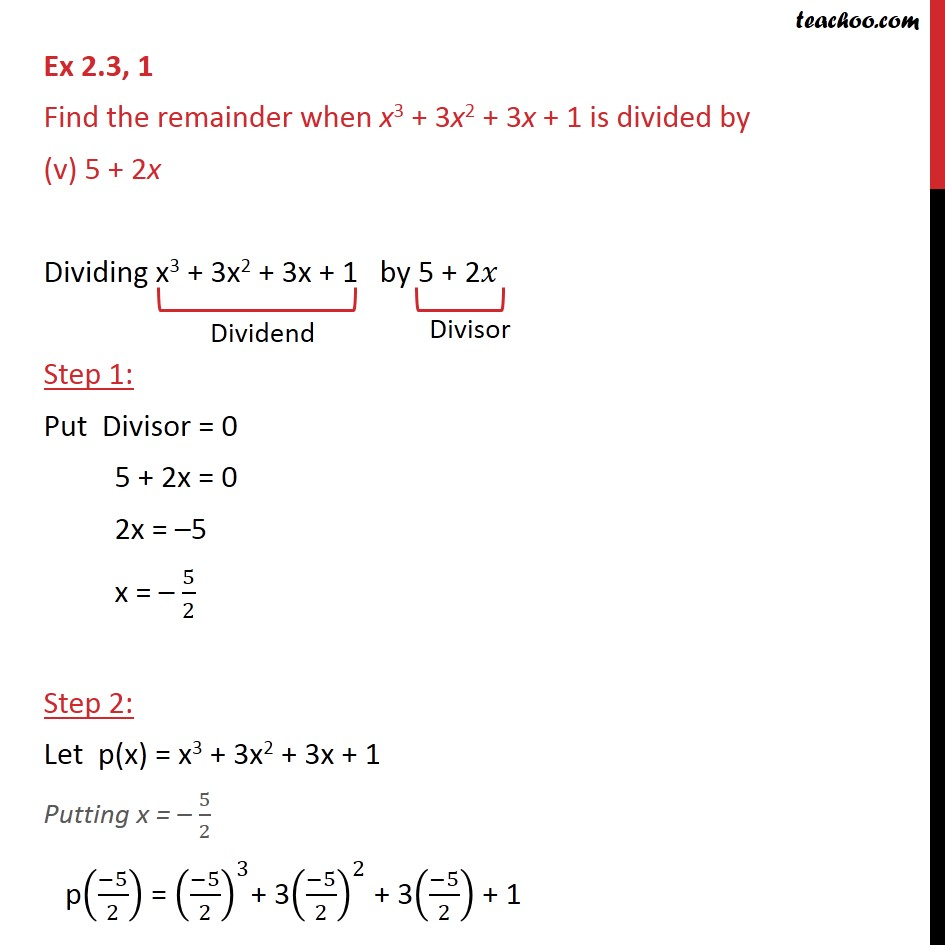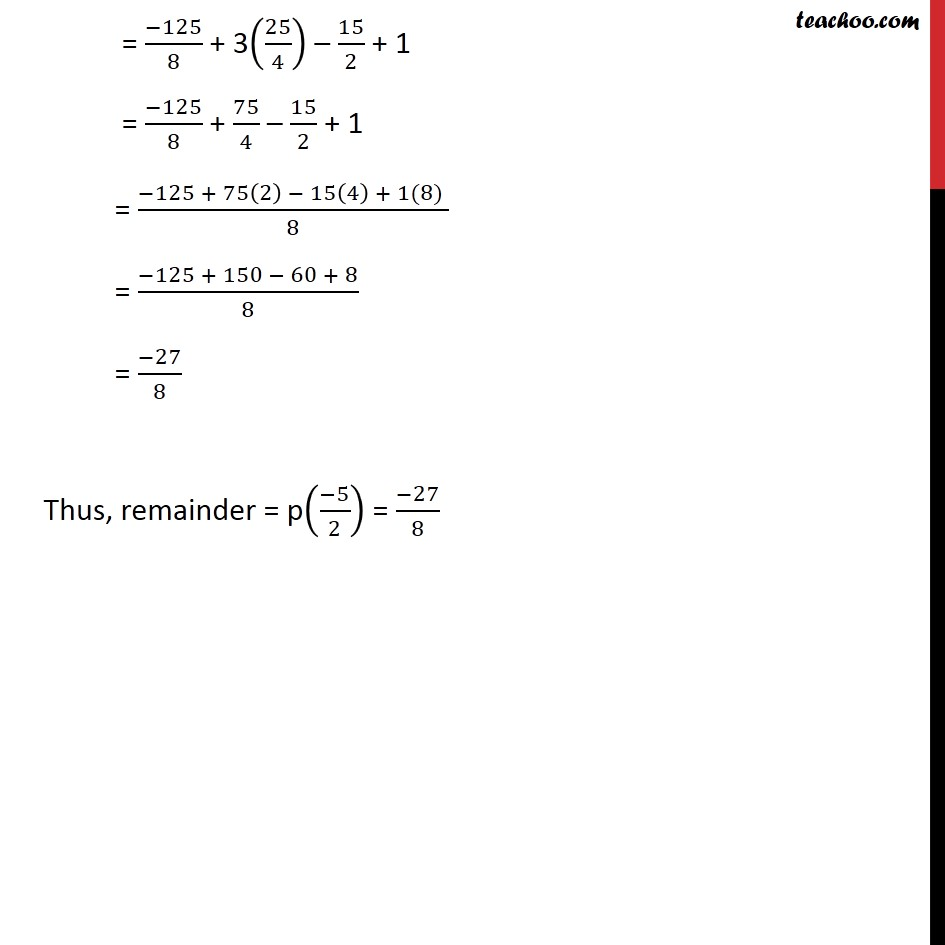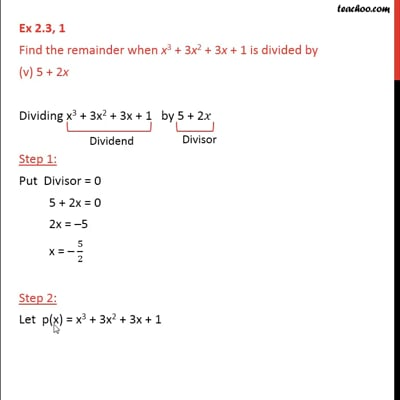Ex 2.3

Chapter 2 Class 9 Polynomials (Term 2)
Serial order wiseThis video is only available for Teachoo black users

### Transcript

Ex 2.3, 1 Find the remainder when x3 + 3x2 + 3x + 1 is divided by (v) 5 + 2x Dividing x3 + 3x2 + 3x + 1 by 5 + 2𝑥 Step 1: Put Divisor = 0 5 + 2x = 0 2x = –5 x = – 5/2 Step 2: Let p(x) = x3 + 3x2 + 3x + 1 Putting x = – 5/2 p((−5)/2) = ((−5)/2)^3+ 3((−5)/2)^2 + 3((−5)/2) + 1 = (−125)/8 + 3(25/4) – 15/2 + 1 = (−125)/8 + 75/4 – 15/2 + 1 = (−125 + 75(2) − 15(4) + 1(8) )/8 = (−125 + 150 − 60 + 8)/8 = (−27)/8 Thus, remainder = p((−5)/2) = (−27)/8